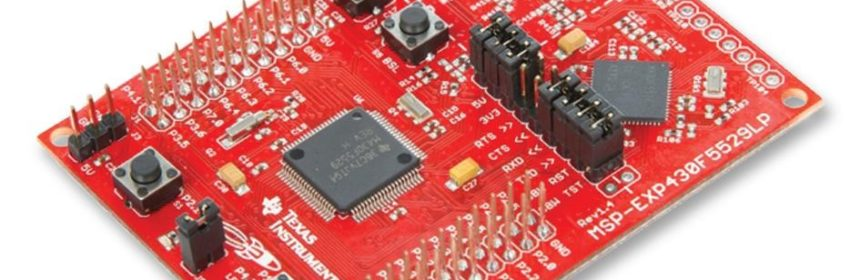## Tinkering TI MSP430F5529## Timer as Counter – TA2

When the clock source of a timer is both regular and repetitive, the timer can be used for tracking/keeping time. When the same timer is fed with an irregular source, the timer acts like a counter. In both cases, pulses are counted – one in synchronous while the other is asynchronous. We can make software-based counters by incrementing/decrementing variables. However, we can do this on hardware level if we use timers as counters and feed them with external inputs.

### Code Example

```#include "driverlib.h"
#include "delay.h"
#include "lcd.h"
#include "lcd_print.h"

void clock_init(void);
void GPIO_init(void);
void timer_T2A3_init(void);

void main(void)
{
signed int value = 0;

WDT_A_hold(WDT_A_BASE);

clock_init();
GPIO_init();
timer_T2A3_init();
LCD_init();

LCD_clear_home();

LCD_goto(1, 0);
LCD_putstr("TA2 as Counter");

LCD_goto(0, 1);
LCD_putstr("Count:");

while(true)
{
value = Timer_A_getCounterValue(TIMER_A2_BASE);
print_C(12, 1, value);

if((value > 20) && (value  40) && (value  60) && (value < 120))
{
GPIO_setOutputHighOnPin(GPIO_PORT_P1,
GPIO_PIN0);

GPIO_setOutputHighOnPin(GPIO_PORT_P4,
GPIO_PIN7);
}

else
{
GPIO_setOutputLowOnPin(GPIO_PORT_P1,
GPIO_PIN0);

GPIO_setOutputLowOnPin(GPIO_PORT_P4,
GPIO_PIN7);
}
};
}

void clock_init(void)
{
PMM_setVCore(PMM_CORE_LEVEL_3);

GPIO_setAsPeripheralModuleFunctionInputPin(GPIO_PORT_P5,
(GPIO_PIN4 | GPIO_PIN2));

GPIO_setAsPeripheralModuleFunctionOutputPin(GPIO_PORT_P5,
(GPIO_PIN5 | GPIO_PIN3));

UCS_setExternalClockSource(XT1_FREQ,
XT2_FREQ);

UCS_turnOnXT2(UCS_XT2_DRIVE_4MHZ_8MHZ);

UCS_turnOnLFXT1(UCS_XT1_DRIVE_0,
UCS_XCAP_3);

UCS_initClockSignal(UCS_MCLK,
UCS_XT2CLK_SELECT,
UCS_CLOCK_DIVIDER_1);

UCS_initClockSignal(UCS_SMCLK,
UCS_XT2CLK_SELECT,
UCS_CLOCK_DIVIDER_4);

UCS_initClockSignal(UCS_ACLK,
UCS_XT1CLK_SELECT,
UCS_CLOCK_DIVIDER_1);
}

void GPIO_init(void)
{
GPIO_setAsPeripheralModuleFunctionInputPin(GPIO_PORT_P2,
GPIO_PIN2);

GPIO_setAsOutputPin(GPIO_PORT_P1,
GPIO_PIN0);

GPIO_setAsOutputPin(GPIO_PORT_P4,
GPIO_PIN7);
}

void timer_T2A3_init(void)
{
Timer_A_initUpModeParam UpModeParam = {0};

UpModeParam.clockSource = TIMER_A_CLOCKSOURCE_EXTERNAL_TXCLK;
UpModeParam.clockSourceDivider = TIMER_A_CLOCKSOURCE_DIVIDER_1;
UpModeParam.timerPeriod = 120;
UpModeParam.timerInterruptEnable_TAIE = TIMER_A_TAIE_INTERRUPT_DISABLE;
UpModeParam.captureCompareInterruptEnable_CCR0_CCIE = TIMER_A_CCIE_CCR0_INTERRUPT_DISABLE;
UpModeParam.timerClear = TIMER_A_SKIP_CLEAR;
UpModeParam.startTimer = true;

Timer_A_stop(TIMER_A2_BASE);

Timer_A_clearTimerInterrupt(TIMER_A2_BASE);

Timer_A_initUpMode(TIMER_A2_BASE,
&UpModeParam);
}```

### Explanation

Here timer T2A3 is used to count incoming pulses. Since counting pulses involves timer’s external input pin, this input pin must be declared as an Input Peripheral Module Function pin.

``GPIO_setAsPeripheralModuleFunctionInputPin(GPIO_PORT_P2, GPIO_PIN2);``

Timer TA2 is setup as follows:

```void timer_T2A3_init(void)
{
Timer_A_initUpModeParam UpModeParam = {0};

UpModeParam.clockSource = TIMER_A_CLOCKSOURCE_EXTERNAL_TXCLK;
UpModeParam.clockSourceDivider = TIMER_A_CLOCKSOURCE_DIVIDER_1;
UpModeParam.timerPeriod = 120;
UpModeParam.timerInterruptEnable_TAIE = TIMER_A_TAIE_INTERRUPT_DISABLE;
UpModeParam.captureCompareInterruptEnable_CCR0_CCIE =                         TIMER_A_CCIE_CCR0_INTERRUPT_DISABLE;
UpModeParam.timerClear = TIMER_A_SKIP_CLEAR;
UpModeParam.startTimer = true;

Timer_A_stop(TIMER_A2_BASE);

Timer_A_clearTimerInterrupt(TIMER_A2_BASE);

Timer_A_initUpMode(TIMER_A2_BASE, &UpModeParam);
}```

Clock source is the very first thing to note in this example. ACLK or SMCLK is not used. Externally clock pulses are applied via timer input pin. Note however timer input pin is also not a CC channel pin.

Up mode counting is used with a top value of 120 and so the timer will count from 0 to 120 and then roll over.

Interrupts are avoided and so polling method is used.

Initially, the timer is kept in halt state and timer settings are not cleared because no setting was applied beforehand.

After setting up the timer, the timer is started but counting doesn’t start until there is a logic transition in the timer’s external input pin. Each incoming pulse increases the timer’s count.

In the main loop, timer’s count is checked or polled on each loop passes. The value of timer count is displayed on an LCD and -LEDs are flashed according to count values.

```value = Timer_A_getCounterValue(TIMER_A2_BASE);
print_C(12, 1, value);

if((value > 20) && (value  40) && (value  60) && (value < 120))
{
GPIO_setOutputHighOnPin(GPIO_PORT_P1, GPIO_PIN0);

GPIO_setOutputHighOnPin(GPIO_PORT_P4, GPIO_PIN7);
}

else
{
GPIO_setOutputLowOnPin(GPIO_PORT_P1, GPIO_PIN0);

GPIO_setOutputLowOnPin(GPIO_PORT_P4, GPIO_PIN7);
}```

### Related Posts

• Pingback: Tinkering TI MSP430F5529 – gStore

• Surya k sHello
I am doing project of msp430g2553 interface(using i2c communication) with temp 100(temperature sensor) and try to read the temperature in dispaly(16*2) but didn’t get the out put (using code composer studio) can u share me any example code for this project

Thank you sir,

•Which sensor? Did you use pullup resistors for SDA-SCL pins?

• CristianWhere is lcd_print.h?

•You want the truth? TI makes and sell “underpowered micros”, you know? Low everything, not only the power but also peripherals. So the price is not justified.

Otherwise, if I’ll move there, I’ll introduce them to my small hobby projects – there are still some advantages.

•I may even make a visual configuration tool of my own for them…

•Yeah the prices of TI products are higher than other manufacturers but I don’t think the hardware peripherals are inferior.

•Not inferior but in not enough numbers compared to STM32.

•True